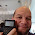## Saturday, July 31, 2021

### HP 48G: Truth Plots

HP 48G: Truth PlotsCalculators Featured:  HP 48G, HP 48G+, HP 48GX

Truth Plots

On the HP 48G, a truth plot tests an inequality or equality for each point of a Cartesian grid.   If the statement is true, the pixel is plotted.  If not, the pixel remains unchanged.  Since each pixel is being tested, a truth plot does take some time to plot.

Example: The inequality x + y ≤ 2, plots a pixel every time inequality is true.

To access a truth plot on the HP 48G:

1.  Press [ right shift ], [ 8 ] (PLOT).

2.  The expression is stored in EQ.  Default:  X is the independent variable and Y is the dependent variable.

3.  Make any necessary adjustments.  If you have to reset your parameters, press [ left shift ], [ 8 ] (PLOT), (PPAR), (RESET).

Truth plots are not unique to the HP 48G series: the current HP Prime can easily and quickly execute truth plots.  The truth plots are renamed "Advanced Graphing App".

Here are examples of truth plots, with the HP 48GX is one the left, the HP Prime is on the right.  The window is set up as x = [-5, 5] and y = [-5,5 ].

x^2 + y^2 ≥ 5(x^3 - y^2) mod 5 ≥ 3sin(x*y) < 3*cos(x*y)Eddie

All original content copyright, © 2011-2021.  Edward Shore.   Unauthorized use and/or unauthorized distribution for commercial purposes without express and written permission from the author is strictly prohibited.  This blog entry may be distributed for noncommercial purposes, provided that full credit is given to the author.

1.1.